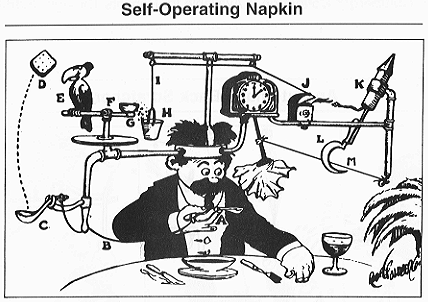# Random Exchange Models

Random Exchange Game

A recent random conversation got me to thinking about random exchange models in economics.

Consider the following game. N people start off with a total amount of money M, so m = M/N per person. Moves are made according the cadence set by the game clock. At each move two players are selected at random. Player A transfers an amount of money m to player B. Unless, of course A is broke: no debt in this game!

The question is: what happens in the long run?

I had misremembered the game, thinking that one player would accumulate all or almost all of the money. The actual outcome is much more interesting. In the long run, the distribution of wealth converges to what is known in physics as the Boltzmann-Gibbs distribution:

The probability of having an amount of money m is proportonal to exp(-m/T) for some constant T proportional to the average amount of money per person in the economy. Why the connection with physics? Boltzman and Gibbs found that in an ensemble of interacting particles obeying certain rules, the probability of being in energy state E is proportional to exp(-E/kT) where T is the absolute temperature and k is Boltzmann’s constant. The absolute temperature scale K is like the centigrade scale C, except that the zero point is -273.16 degrees C. Very cold!

The standard example of the Boltzmann-Gibbs distribution is an ideal gas.

Having remembered the rules of the game, I decided to make a little game that I could play with. And think about. How fast does the initial distribution of money change from perfect equality to a stationary distribution? (A distribution that does not change with time, even though stuff still happens, i.e., money is still exchanged.) What would be the effects of taxation? How does the Gini coefficient (a measure of inequality) change with time and with change of tax policy? Etc.

Below is a screenshot of the game, written using Evan’s Elm Playground. You will find the game at jxxcarlson.github.io. The code is at github.com/jxxcarlson/small-economy.

The random exchange model is, of course a radically simple (and also controversial) model. Nonetheless, it is one that we can learn from. It is often better to have a simple model whose principles are clear that we can compare with reality and argue about intelligently than a very complicated model whose working principles are hard to reason about.Note. While the theory of the random exchange model is subject to debate, its agreement with real income or wealth data is excellent — except for the “fat tails” of very high income/wealth. For the tails, the data fit a power law, something observed many years ago by Pareto (1848-1923) and discussed more recently in Yakovlenko 2022. See also Gosh et al, Biswas et al

As a recovering academic, I could go on and on about this, but I’ll leave further discussion to the comments.3 Likes

How did you decide on the radius of the circles to be `max 1 (person.capital ^ 2.5 / 500)`? The most intuitive visualization to me would be that the area of the circle is proportional to the capital.

Exponent 2 is a natural choice (think of coins), but exponent 3 is also a candidate, since a fixed amount of money buys a fixed number of liters of milk or gasoline, for example. But exponent 3 did not work out visually: the disks grew to rapidly in size.

I would expect the exponent to be 1/2 to make the area proportional to the value, or 1/3 if we’re simulating volume, rather than 2 and 3.

You are correct. My thinking was based on my guess of how the user perceives the disks. As an example, suppose we use

``````radius =
max 1 (250 * person.capital ^ 0.5 / 500)
``````

Below is what things look like after 80,000 transactiions when there is already a great deal of inequality (q5/q1 = 60, gini = 0.51, fairly high). But we don’t perceive much difference. So my guess is that we are more sensitive to the angular diameter. However, I really don’t know. I mostly took an experimental appraoach to this.

One more thought on this. Suppose that we agree that angular diameter is what we are most sensitive to, and also that we want the impact on our nervous system to be proportional to the amount of money for each person. Then the radius of each person’s disk should be proportional to the amount of money. Then something like this could work:

``````  radius = max 1 ( person.capital ^ 1.0 / 5)
``````

gives pretty good results. Here is what things look at t = 0:

And here is what the state of the system is at t = 25,000 with q5/q1 = 60:

So (a) all dots look small at t = 0, (b) there is good visual contrast between q5 and q1 at t = 25,000.

Thanks for bring this question up!

This topic was automatically closed 10 days after the last reply. New replies are no longer allowed.